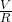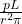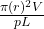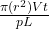## A potential difference of 3.00 nV is set up across a 2.00 cm length of copper wire that has a radius of 2.00 mm. How much charge drifts thro

Question

A potential difference of 3.00 nV is set up across a 2.00 cm length of copper wire that has a radius of 2.00 mm. How much charge drifts through a cross section in 3.00 ms

in progress 0
4 months 2021-09-05T15:42:55+00:00 1 Answers 53 views 0

1. The number of charge drifts are 3.35 X 10⁻⁷C

Explanation:

Given:

Potential difference, V = 3 nV = 3 X 10⁻⁹m

Length of wire, L = 2 cm = 0.02 m

Radius of the wire, r = 2 mm = 2 X 10⁻³m

Cross section, 3 ms

charge drifts, q = ?

We know,

the charge drifts through the copper wire is given by

q = iΔt

where Δt = 3 X 10⁻³s

and i =where R is the resistance

R =ρ is the resistivity of the copper wire = 1.69 X 10⁻⁸Ωm

So, i =q =Substituting the values,

q = 3.14 X (0.02)² X 3 X 10⁻⁹ X 3 X 10⁻³ / 1.69 X 10⁻⁸ X 0.02

q = 3.35 X 10⁻⁷C

Therefore, the number of charge drifts are 3.35 X 10⁻⁷C